﻿ 一种改进的分形图像编码方法 An Improved Fractal Image Coding Method

Computer Science and Application
Vol.07 No.11(2017), Article ID:22761,7 pages
10.12677/CSA.2017.711119

An Improved Fractal Image Coding Method

Lingyun Qi

College of Computer Science, Sichuan University, Chengdu Sichuan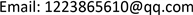Received: Nov. 3rd, 2017; accepted: Nov. 16th, 2017; published: Nov. 23rd, 2017ABSTRACT

The traditional image coding method has become the general format; development potential is not great. The method of fractal image coding compression method is a novel idea; it has great potential for development. Based on the theory of fractal coding and from coding time, compression ratio and the decompression effect three aspects, we make research and improvement on Jacquin’s basic fractal coding method, and propose an improved fractal image coding method. Experimental results show that compared to the Jacquin’s basic fractal coding method, the method in this paper is improved on the image compression time, compression ratio and peak signal to noise ratio.

Keywords:Fractal Image Coding, Compression Ratio, Decompression EffectCopyright © 2017 by author and Hans Publishers Inc.1. 引言

2. 基本分形编码方法

1981年，J.E.Hutchinson将迭代函数理论(Iterated Function Theory)引入分形理论中  。1989年，Barnsley最先将分形理论运用在图像压缩上，提出了基于迭代函数系统(IFS，即Iterated Function System)的分形图像压缩方法，并对特定的几幅图像实现了高达10,000:1的压缩比  。该方法从图像的结构和人眼的视觉角度对图像进行压缩，打破了传统的熵压缩方法的理论极限，受到人们的高度关注，成为国内外学者的研究热点   。但是该方法中的图像分割需要专业的人员进行人机交互操作，不能自动实现。

1990年，Barnsley的学生Jacquin发表博士论文，第一次提出局部函数迭代系统  (LIFS，即Local Iterated Function system)，实现了第一个具有实际意义的全自动分形编码方法。该方法是基于图像块的压缩方法，使用局部块的仿射变换表示全局的仿射变换。Jacquin基本分形编码方法将待压缩图像分割成多个值域块和定义域块。对每个定义域块进行四邻域像素平均算法收缩为值域块大小，并通过8种变换(包括4个旋转，4个反射)形成8个子块，所有的这些子块就组成码本或虚拟码书。然后对每个值域块在虚拟码本中寻找其最佳匹配块。编码后的分形码包括5个参数，分别是对比度调节s、亮度偏移量o、位置参数y、x以及变换参数m。其解码是用迭代因子作用于任何初始图像迭代形成解压图像。

3. 改进的分形编码方法

3.1. 编码方法

1、设输入图像I，图像大小为N × N。将图像I分割成互不相交的B × B大小的值域块Ri，且所有值域块的并集为原图像。即分割满足公式(1)。

$I=\underset{i=1}{\overset{N/B×N/B}{\cup }}{R}_{i}$ ，且 ${R}_{i}\cap {R}_{j}=\Phi \left(i\ne j\right)$ (1)

2、将图像I分割成可以相交的F × F大小的定义域块Di，其中F = 2 × B。然后对每个定义域D块进行4邻域像素均值算法收缩为B × B大小的像素块，这些像素块组成“虚拟码本”。

3、在虚拟码本内，对每个值域块Ri寻找其最佳匹配块Dbest，使得块Ri可由最佳匹配块Dbest通过亮度变换来近似，即有 ${R}_{i}\approx s\cdot {D}_{best}+o\cdot 1$ ，其中1是亮度值均为1的常值块，s代表对比度收缩因子，o代表亮度偏移量。最佳匹配块Dbest的寻找需满足公式(2)，即满足Ri与Dbest的仿射变换间的差异最小。公式(2)也可表示为公式(3)，其中 $E\left(R,D\right)$ 表示最小平方误差。公式(3)是关于s、o的二元多项式的最小值问题，由最小二乘法可以得到s、o的值。最后将仿射变换参数s，o以及最佳匹配块的位置参数dx，dy记录在矩阵中，即完成对一个值域块的编码。遍历图像中所有值域块，完成图像编码。

$\underset{j}{\mathrm{min}}\left\{\underset{s,o\in R}{\mathrm{min}}‖{R}_{i}-\left(s\cdot {D}_{j}+o\cdot 1\right)‖\right\}=\underset{s,o\in R}{\mathrm{min}}{R}_{i}-\left({s}_{i}\cdot {D}_{best}+{o}_{i}\cdot E\right)$ (2)

$E\left(R,D\right)=\underset{s,o\in R}{\mathrm{min}}{‖R-\left(s\cdot D+o\cdot 1\right)‖}^{2}$ (3)

4、使用基本分形解压算法得到解压图像 ${I}^{\prime }$ ，并计算误差 $\Delta =I-{I}^{\prime }$ 。对误差值按递增的顺序进行排序，形成一个数列，并计算该数列的上四分位数Qu、中位数Qm、下四分位数Ql、上限 $\text{Max}=Qu+1.5\left(Qu-Ql\right)$ 、下限 $\text{Min}=\text{max}\left\{0,\text{\hspace{0.17em}}Ql-1.5\left(Qu-Ql\right)\right\}$ 以及误差绝对值的最大值M。其中上四分位数Qu为居于数列四分之一位置处的误差数据值，中位数Qm为居于数列中间位置处的误差数据值，下四分位数Ql为居于数列四分之三位置处的误差数据值。

5、对误差值进行区域划分并进行映射。运用分类统计思想，我们对误差进行区域划分。将绝对值处在 $\left\{0,\text{\hspace{0.17em}}\text{Max}\right\}$ 间的误差值映射为0，不进行存储。绝对值在 $\left\{\text{Max},\text{\hspace{0.17em}}+\infty \right\}$ 间的误差分为14个区域，并映射到数字1至14，这样只用4 bit即可将误差值保存，其中用奇数值表示正值误差，偶数值表示负值误差。又因为要将同一区域内的所有点视为相同类型点，并以该区域均值代替区域内所有误差点的值，故为了保证解压图像的PSNR值，区域间隔的选取很重要，不能过大。本文区域间隔S的取值计算方法为 $S=\left(M-\text{Max}\right)/7$ 。因此， $\left\{\text{Max}+S×\left(i-1\right),\text{\hspace{0.17em}}\text{Max}+S×i\right\}$ 区域误差映射为数字 $2×i-1\left(i=1~6\right)$$\left\{-\text{Max}-S×i,\text{\hspace{0.17em}}-\text{Max}-S×\left(i-1\right)\right\}$ 区域误差映射为数字 $2×i-1\left(i=1~6\right)$$\left\{\text{Max}+S×6,\text{\hspace{0.17em}}+\infty \right\}$ 区域误差映射为数字13， $\left\{-\infty ,\text{\hspace{0.17em}}-\text{Max}-S×6\right\}$ 区域误差映射为数字14。求每个区域的误差均值，设为 $mea{n}_{i}$ ，其中 $i=1~14$ 。将映射关系(i, $mea{n}_{i}$ )存入编码文件中。

6、对误差进行存储。误差的存储分为两部分。一部分是高频信息存储，选择误差最大值存储。另一部分是低频信息存储，将误差图像分为2 × 2的矩阵，对矩阵中值小于4的所有误差点求均值，并以2 bit进行存储。最后将编码数据以.mat文件输出。

3.2. 解码方法

1、输入编码文件F。读取编码信息并计算出原图像尺寸。

2、定义原图像大小的单位矩阵作为初始图像，设为H。同压缩过程一样将图像分割成相同大小的值域块。对于每个值域块Ri依据编码数据中的位置信息找到相应的最佳匹配块 ${D}_{best}$ ，对 ${D}_{best}$ 块进行4邻域像素均值收缩算法和仿射变换操作，最后将结果赋值给该Ri块。对所有值域块R操作完成后，经过拼贴就完成了图像的一次迭代，设此时图像为 ${H}^{\prime }$

3、判断是否达到预先设定的迭代次数，若已经达到预先设定的迭代次数，则执行步骤4，否则就以图像 ${H}^{\prime }$ 作为初始图像，再次执行步骤2来继续完成图像迭代。本文中设定的迭代次数为6。迭代次数的设定选择要综合考虑解压效果和解压时间两个因素。通过对多幅图像进行不同迭代次数选择的实验，发现迭代次数达到6时，对图像的解压效果不再有明显的增强效果。

4、提取编码中存储的误差信息以及误差映射信息。依据压缩方法中误差存储的规则，反向得出误差信息。再将误差加入图像 ${H}^{\prime }$ 中，完成解码。最后输出解压图像 ${H}^{\prime }$

4. 仿真实验结果

$\text{MSE}=\frac{1}{YX}\sum _{1}^{Y}\sum _{1}^{X}{\left({f}^{\prime }\left(i,j\right)-f\left(i,j\right)\right)}^{2}$ (4)

$\text{PSNR}=10\mathrm{log}\frac{{255}^{2}}{\text{MSE}}$ (5)Figure 1. Lena diagram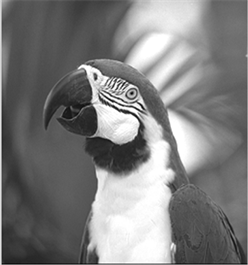Figure 2. Bird diagram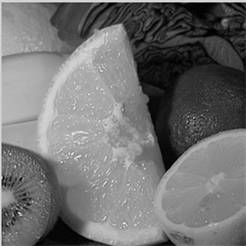Figure 3. Fruits diagram(a)(b)

Figure 4. Decompression effect of lena diagram using the method and the basic fractal method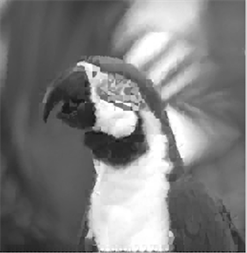(a)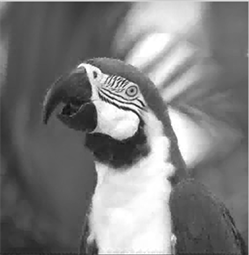(b)

Figure 5. Decompression effect of bird diagram using the method and the basic fractal method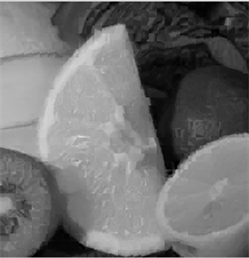(a)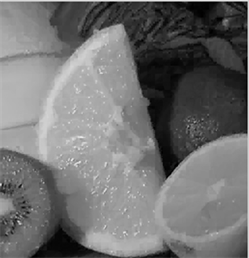(b)

Figure 6. Decompression effect of fruits diagram using the method and the basic fractal methodTable 1. Experimental results using the improved coding method and the basic fractal coding method

5. 小结

An Improved Fractal Image Coding Method[J]. 计算机科学与应用, 2017, 07(11): 1052-1058. http://dx.doi.org/10.12677/CSA.2017.711119

1. 1. Wikipedia Fractal Compression. https://en.wikipedia.org/wiki/Fractal_compression#cite_ref-18

2. 2. Mandelbrot (1982) The Fractal Geometry of Nature. Freeman W H, Mandelbrot, 466.

3. 3. Hutchinson, J.E. (1981) Fractals and Self-Similarity. Indiana University Mathematics Journal, 3, 713-747. https://doi.org/10.1512/iumj.1981.30.30055

4. 4. Barnsley, M.F. (1988) A Better Way to Compress Image. Byte Magazine, 1, 215-223.

5. 5. Cabrelli, C.A., Falsetti, M.C. and Molter, U.M. (2002) Fractal Block-Coding: A Functional Approach for Image and Signal Processing. Computers and Mathematics with Applications, 44, 1183-1200. https://doi.org/10.1016/S0898-1221(02)00225-0

6. 6. Kima, T., Van Dyck, R.E. and Miller, D.J. (2002) Hybrid Fractal Zero Tree Wavelet Image Coding. Signal Processing: Image Communication, 17, 347-360. https://doi.org/10.1016/S0923-5965(02)00003-6

7. 7. Jacquin, A.E. (1992) Image Coding Based on a Fractal Theory of Iterated Contractive Image Transformations. IEEE Trans ImageProcess, 1, 18-30. https://doi.org/10.1109/83.128028

8. 8. 王惠溧. 基于三角形分割的分形图像压缩算法[J]. 科技向导, 2013(36): 278.

9. 9. Tan, T. and Yan, H. (2002) The Fractal Neighbor Distance Measure. Pattern Recognition, 35, 1371-1387.

10. 10. 蔡芳, 徐乃平, 孙隆和. 快速分形图像压缩算法[J]. 西北工业大学学报, 1999, 17(3): 355-359.

11. 11. 陈家琪, 唐元刚. 小波与分形混合图像压缩编码[J]. 计算机与信息技术, 2007(35): 272-274.